Raspberry Pi

# How to Make an Obstacle-Avoiding Robot With Raspberry PiJune 15, 2017 by Vikas Kumar
Share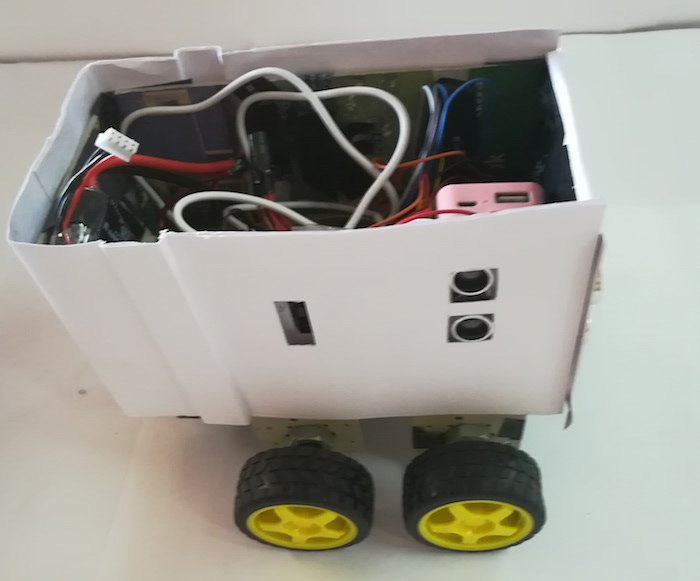Use a Raspberry Pi to make this simple obstacle-avoiding robot.

## Hardware

 1 110090141 1 SEN-15569 1 L298N 1 MIKROE-192

When my project mates and I started our B. Tech project on an â€œAutonomous Mobile Robot Navigation Using Deep Reinforcement Learningâ€, we couldnâ€™t find a complete circuit diagram of aÂ robot that uses a Raspberry Pi. All we could find was connection diagrams of various components with a Raspberry Pi individually. So we thought we'd write this article after completing our project.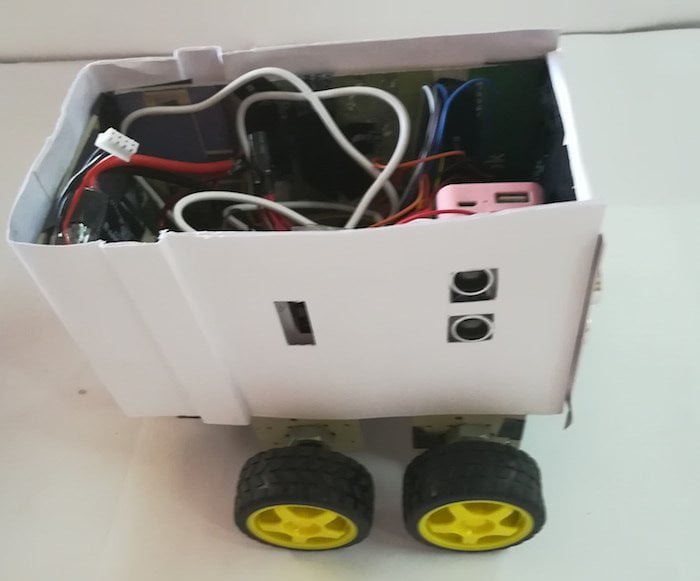### How Does the Raspberry Pi Robot Work?

The Raspberry Pi has the ability to interact with the outside world using its GPIO pins, USB, Ethernet, HDMI, LCD, and camera. Here we are using Raspberry Pi 3 which has a 64bit ARMv7 Quad Core Processor having 1 GB RAM along with onboard Wi-Fi and Bluetooth.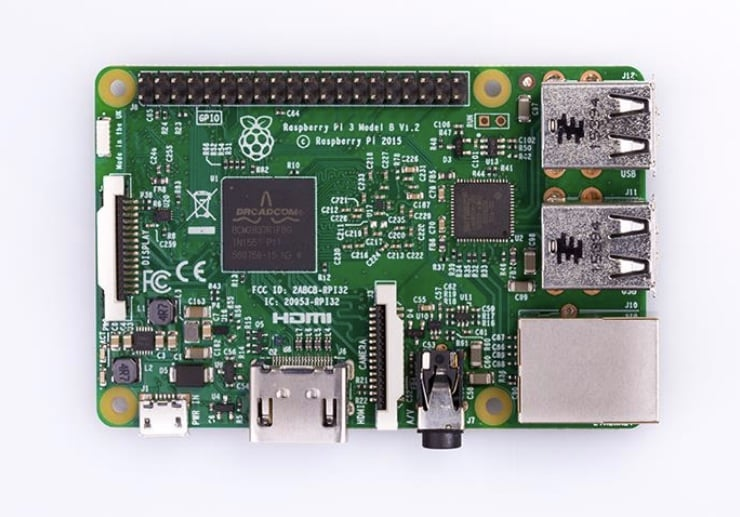The ultrasonic ranging module, HC-SR04 was used in this project. It provides 2cm-400cm non-contact measurement function, the ranging accuracy can reach up to 3mm. This module includes an ultrasonic transmitter, receiver, and control circuit.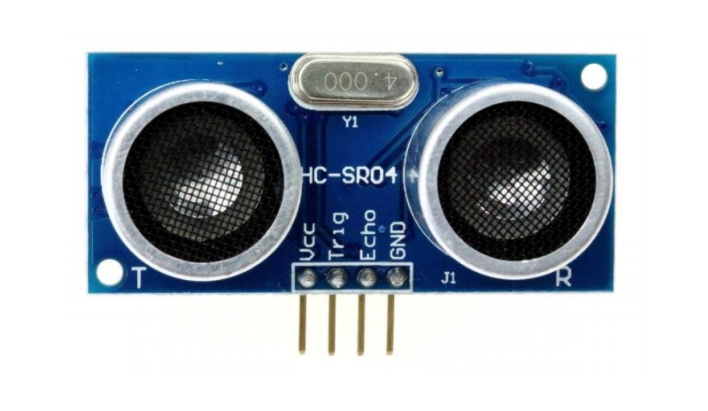An infrared sensor is an electronic instrument which is used to sense certain characteristics of its surroundings by either emitting and/or detecting infrared radiation. Infrared sensors are also capable of measuring the heat being emitted by an object and detecting motion. The distance measuring range is 10 cm to 80 cm.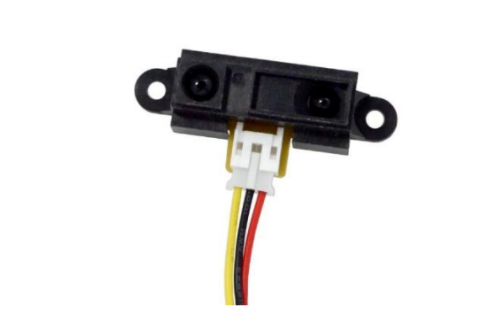The L298N driver module allows DC motor to drive in either direction. It can control a set of two DC motors simultaneously in any direction. This means that we can control two DC motors with a single motor controller. The L298N receives a signal from the Raspberry Pi and transmits the relative signal to the motors. It has two voltage pins, one of which is used to draw current for the L298N and the other is used to apply voltage to the motors. The L298N switches its output signal according to the input received from the microprocessor.The voltage regulator IC maintains the output voltage at a constant value. The 7805 IC, the voltage regulator integrated circuit (IC) is a member of 78xx series of fixed linear voltage regulator ICâ€™s used to maintain such fluctuations. The xx in 78xx indicates the fixed output voltage it provides. The 7805 IC provides +5 volts regulated power supply with provisions to add heat sink as well.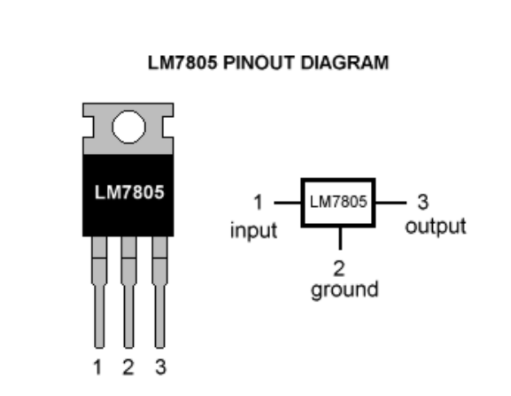The obstacle detection and avoiding robot uses two 200 rpm and 12 V DC geared motors. The motor used has a 6mm shaft diameter with internal holes. The internal holes are for easy mounting of the wheels by using screws. It is an easy to use low-cost motor for robotics application. Two dummy motors are also used along with two DC motors in the robot. A dead axle dummy motor is designed in such a way that it represents the axle size and shape of an actual motor but is a passive component as it is just used as a support for fixing non powered wheels in the robot chassis.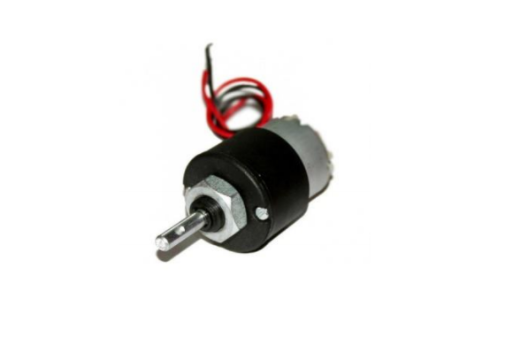### Circuit Diagram

In the circuit diagram, solid lines represent live wires (Vcc), dashed lines represent ground and the dotted lines represent the wires through which information is transferred from the Raspberry Pi to the actuators and vice versa.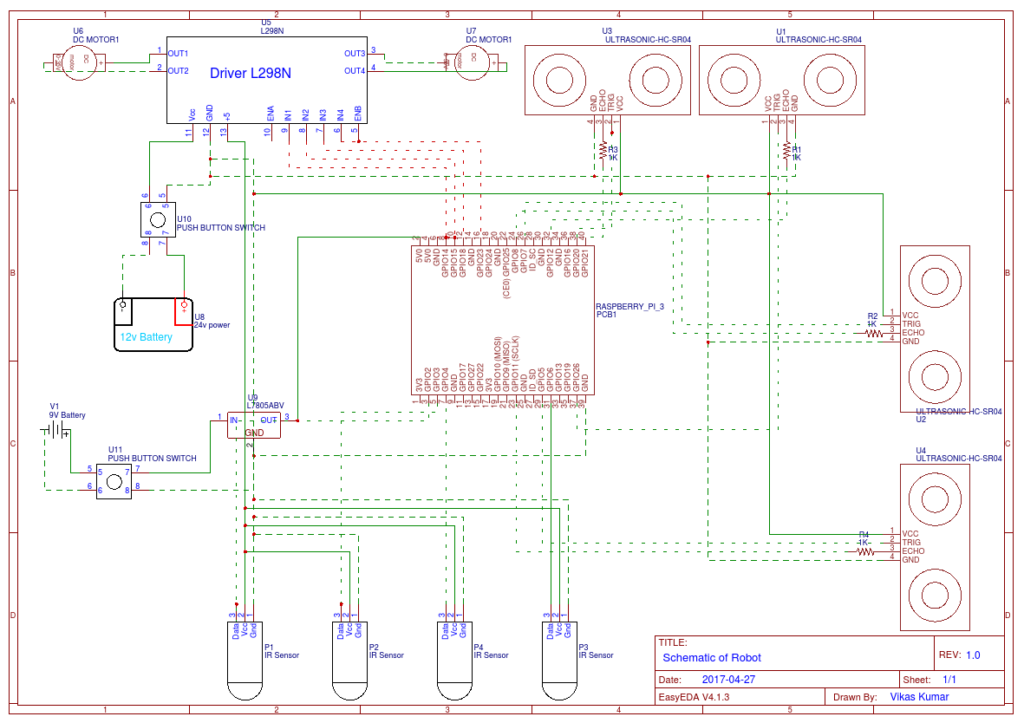View full-size schematic.

Here is a clip of our robot in action! You can also find the codes needed to make your own Raspberry Pi obstacle avoiding robot below in written and downloadable form.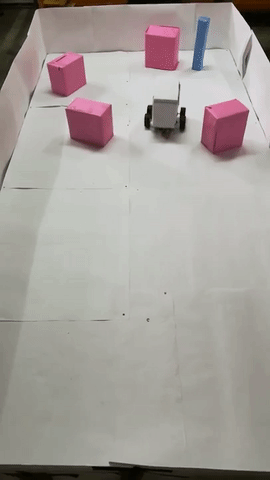### Programming: Manual Motion

```import curses

import RPi.GPIO as GPIO```
```class MotorControler(object):

def __init__(self, parent=None):

self._data = {'name': 'MOTOR', 'delay': 0.01, 'LD': 14, 'LU': 15, 'RD': 18, 'RU': 23}

self.init_pin()

def init_pin(self):

self.GPIO_LD_PIN = self._data.get('LD', -1) self.GPIO_LU_PIN = self._data.get('LU', -1)

self.GPIO_RD_PIN = self._data.get('RD', -1) self.GPIO_RU_PIN = self._data.get('RU', -1)

if self.GPIO_LD_PIN == -1 or self.GPIO_LU_PIN == -1 or self.GPIO_RD_PIN == -1 or self.GPIO_RU_PIN == -1:

print('message', 'FATAL ERROR : INVALID PIN ENCOUNTER # ' + str(self.GPIO_LD_PIN) + ', ' + + str(

self.GPIO_LU_PIN) + ', ' + + str(self.GPIO_RD_PIN) + ', ' + + str(self.GPIO_RU_PIN))

# pin setup

# set GPIO numbering mode and define output pins

GPIO.setup(self.GPIO_LD_PIN, GPIO.OUT) GPIO.setup(self.GPIO_LU_PIN, GPIO.OUT) GPIO.setup(self.GPIO_RD_PIN, GPIO.OUT) GPIO.setup(self.GPIO_RU_PIN, GPIO.OUT) time.sleep(0.5) # warmup time self.stop()

def stop(self):

GPIO.output(self.GPIO_LD_PIN, False) GPIO.output(self.GPIO_LU_PIN, False)

GPIO.output(self.GPIO_RD_PIN, False) GPIO.output(self.GPIO_RU_PIN, False)

def step_forward(self):

GPIO.output(self.GPIO_LD_PIN, False) GPIO.output(self.GPIO_LU_PIN, True)

GPIO.output(self.GPIO_RD_PIN, False) GPIO.output(self.GPIO_RU_PIN, True) print('Move Forward')

def step_backward(self):

GPIO.output(self.GPIO_LD_PIN, True) GPIO.output(self.GPIO_LU_PIN, False)

GPIO.output(self.GPIO_RD_PIN, True) GPIO.output(self.GPIO_RU_PIN, False) print('Move Backward')

def step_right(self):

GPIO.output(self.GPIO_LD_PIN, True) GPIO.output(self.GPIO_LU_PIN, False)

GPIO.output(self.GPIO_RD_PIN, False) GPIO.output(self.GPIO_RU_PIN, True) print('Move Right')

def step_left(self):

GPIO.output(self.GPIO_LD_PIN, False) GPIO.output(self.GPIO_LU_PIN, True)

GPIO.output(self.GPIO_RD_PIN, True) GPIO.output(self.GPIO_RU_PIN, False) print('Move Left')

def move_forward(self, count=15):

for i in range(count):

self.step_forward() self.stop()

def move_backward(self, count=15):

for i in range(count):

self.step_backward() self.stop()

def move_right(self, count=15):

for i in range(count):

self.step_right() self.stop()

def move_left(self, count=15): for i in range(count):

self.step_left() self.stop()

#set GPIO numbering mode and define output pins GPIO.setmode(GPIO.BOARD)

# Get the curses window, turn off echoing of keyboard to screen, turn on # instant (no waiting) key response, and use special values for cursor keys screen = curses.initscr() curses.noecho() curses.cbreak() screen.keypad(True) motor = MotorControler() try:

while True:

char = screen.getch() if char == ord('q'):

break elif char == curses.KEY_UP:

motor.move_forward(count=15)

elif char == curses.KEY_DOWN:

motor.move_backward(count=15) elif char == curses.KEY_RIGHT: motor.move_right(count=15) elif char == curses.KEY_LEFT: motor.move_left(count=15) elif char == 10: motor.stop()

finally:

#Close down curses properly, inc turn echo back on!
curses.endwin()
GPIO.cleanup()```

### Programming: Automatic Motion

```import RPi.GPIO as GPIO import time import numpy as np

GPIO.setmode(GPIO.BOARD) GPIO.setwarnings(False)

class MotorControler(object):

def __init__(self, parent=None):

self._data = {'name': 'MOTOR', 'delay': 0.01, 'LD': 14 'LU': 15, 'RD': 18, 'RU': 23}

self.init_pin()

def init_pin(self):

self.GPIO_LD_PIN = self._data.get('LD', -1) self.GPIO_LU_PIN = self._data.get('LU', -1)

self.GPIO_RD_PIN = self._data.get('RD', -1) self.GPIO_RU_PIN = self._data.get('RU', -1)

if self.GPIO_LD_PIN == -1 or self.GPIO_LU_PIN == -1 or self.GPIO_RD_PIN == -1 or self.GPIO_RU_PIN == -1:

print('message', 'FATAL ERROR : INVALID PIN ENCOUNTER # ' + str(self.GPIO_LD_PIN) + ', ' + + str(

self.GPIO_LU_PIN) + ', ' + + str(self.GPIO_RD_PIN) + ', ' + + str(self.GPIO_RU_PIN))

# pin setup

# set GPIO numbering mode and define output pins

GPIO.setup(self.GPIO_LD_PIN, GPIO.OUT) GPIO.setup(self.GPIO_LU_PIN, GPIO.OUT) GPIO.setup(self.GPIO_RD_PIN, GPIO.OUT) GPIO.setup(self.GPIO_RU_PIN, GPIO.OUT) time.sleep(0.5) # warmup time self.stop()

def stop(self):

GPIO.output(self.GPIO_LD_PIN, False) GPIO.output(self.GPIO_LU_PIN, False)

GPIO.output(self.GPIO_RD_PIN, False) GPIO.output(self.GPIO_RU_PIN, False)

def step_forward(self):

GPIO.output(self.GPIO_LD_PIN, False) GPIO.output(self.GPIO_LU_PIN, True)

GPIO.output(self.GPIO_RD_PIN, False) GPIO.output(self.GPIO_RU_PIN, True) print('Move Forward')

def step_backward(self):

GPIO.output(self.GPIO_LD_PIN, True) GPIO.output(self.GPIO_LU_PIN, False)

GPIO.output(self.GPIO_RD_PIN, True) GPIO.output(self.GPIO_RU_PIN, False) print('Move Backward')

def step_right(self):

GPIO.output(self.GPIO_LD_PIN, True) GPIO.output(self.GPIO_LU_PIN, False)

GPIO.output(self.GPIO_RD_PIN, False) GPIO.output(self.GPIO_RU_PIN, True) print('Move Right')

def step_left(self):

GPIO.output(self.GPIO_LD_PIN, False) GPIO.output(self.GPIO_LU_PIN, True)

GPIO.output(self.GPIO_RD_PIN, True) GPIO.output(self.GPIO_RU_PIN, False) print('Move Left')

def move_forward(self, count=15):

for i in range(count):

self.step_forward() self.stop()

def move_backward(self, count=15):

for i in range(count):

self.step_backward() self.stop()

def move_right(self, count=15):

for i in range(count):

self.step_right() self.stop()

def move_left(self, count=15): for i in range(count):

self.step_left() self.stop()

IR_SENSOR_A = 2 IR_SENSOR_B = 3 IR_SENSOR_C = 6 IR_SENSOR_D = 4

GPIO.setup(IR_SENSOR_A, GPIO.IN) GPIO.setup(IR_SENSOR_B, GPIO.IN) GPIO.setup(IR_SENSOR_C, GPIO.IN) GPIO.setup(IR_SENSOR_D, GPIO.IN)

time.sleep(0.5) # warmup time

ir_measure_a = GPIO.input(IR_SENSOR_A) ir_measure_b = GPIO.input(IR_SENSOR_B) ir_measure_c = GPIO.input(IR_SENSOR_C) ir_measure_d = GPIO.input(IR_SENSOR_D)

print(ir_measure_a, ir_measure_b, ir_measure_c, ir_measure_d)

return [ir_measure_a, ir_measure_b, ir_measure_c, ir_measure_d]

# FOR DEMO def run():

motor = MotorControler()

while True:

if sensor == 0 and sensor == 0:

motor.move_forward(count=15) elif sensor == 1 and sensor == 1:

if np.random.ranf() > 0.5 :

motor.move_left(count=15) else:

motor.move_left(count=15) # provide more condotion for better maneuvering

run()```

You can download the demo codes below:
Manual Motion Code
Automatic Motion Code